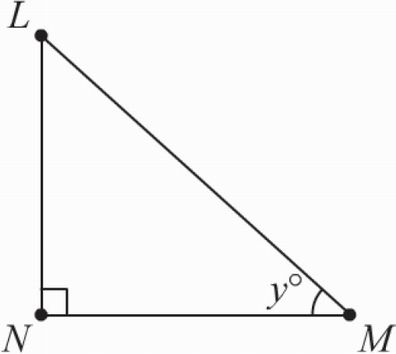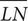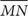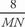# SAT Math Multiple Choice Question 131: Answer and Explanation

### Test Information

Question: 131

11.In the figure above, if y = 40 and= 8, which of the following most closely approximates the length of?

• A. 0.1
• B. 9.53
• C. 10.44
• D. 12.45

B Triangle legs LN and MN are opposite and adjacent, respectively, to ∠y. Therefore, from SOHCAHTOA, we need to use the tangent trigonometric function. Plugging in the values that the question gives us into the equation for tangent, we get tan 40° =. Now, use your calculator to determine that the length of MN most closely approximates 9.53, which is (B).